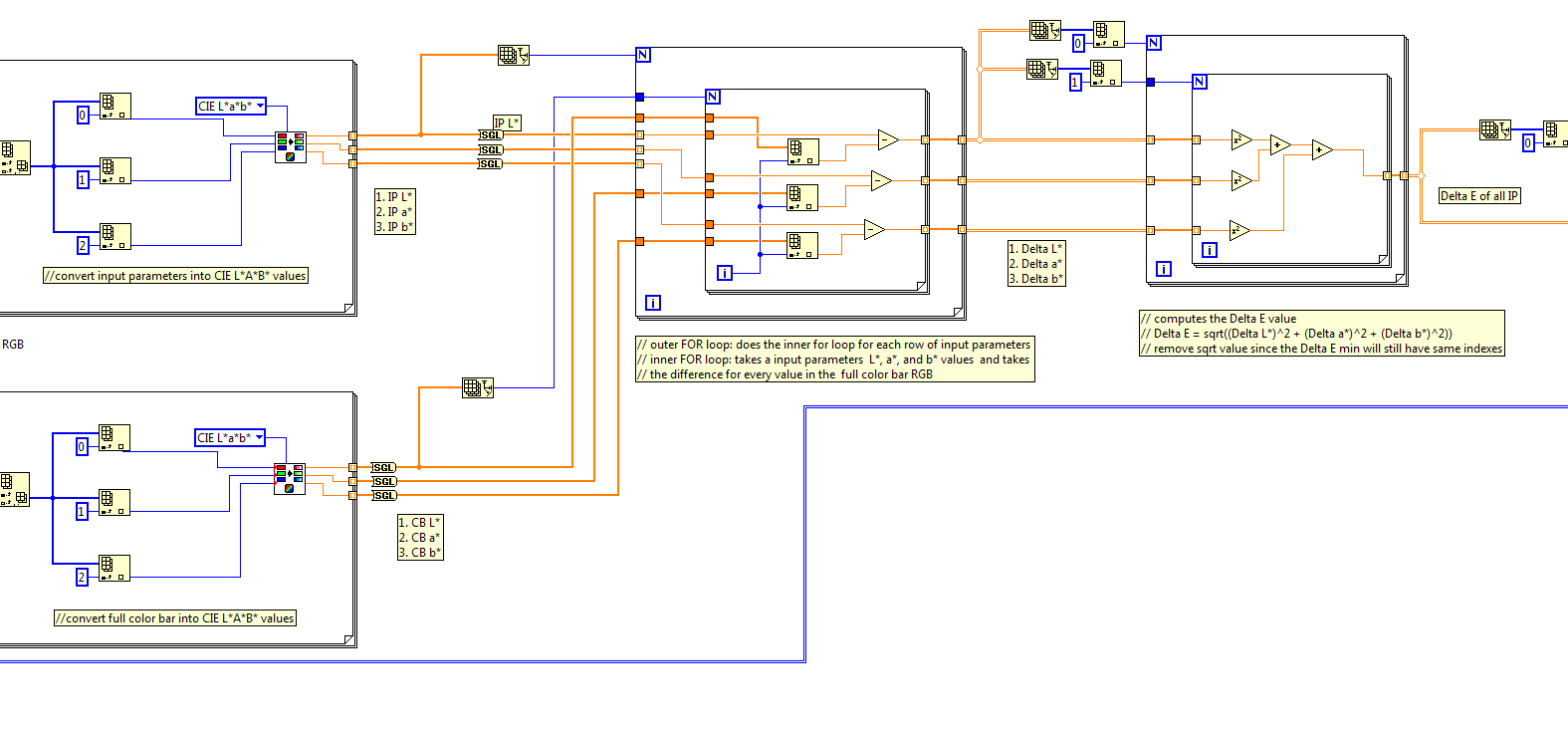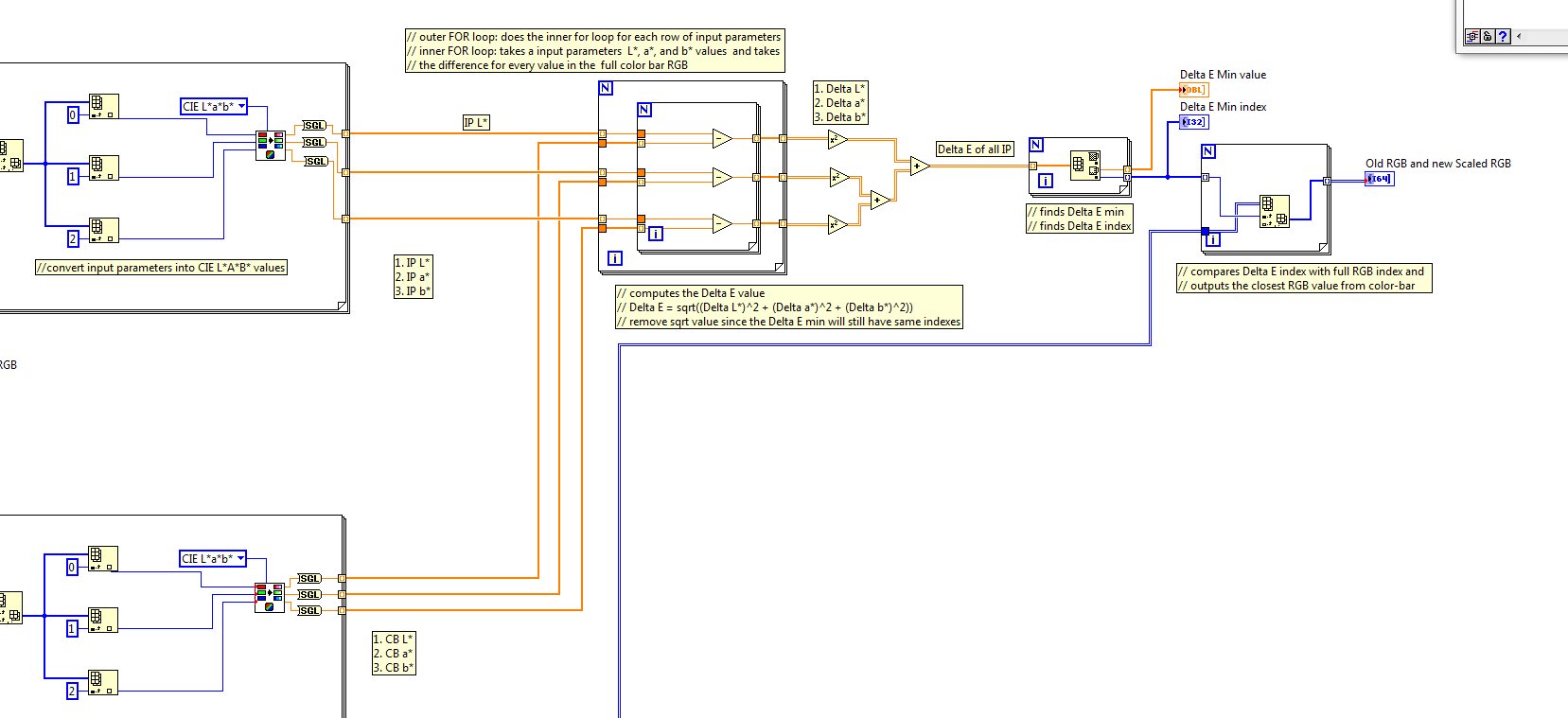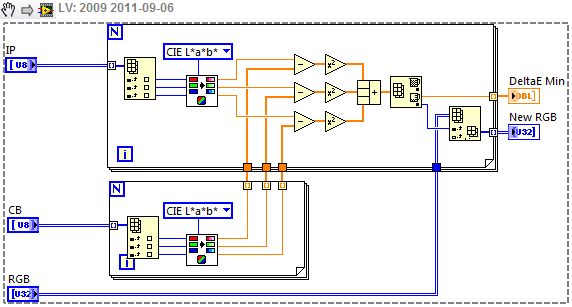# LabVIEW

cancel
Showing results for
Did you mean:

## more efficient memory usage for large arrays

Hello,

This is my first question I have posted on this forum and is relatively simple. (however I can't seem to find an answer that I can understand)

This is a little project I'm trying to do for my uni research lab and was wondering how I can make this portion of my code more efficient (I run out of memory when I run the main VI and it states the problem lies in the portion shown below)

Shown below is a snippet of my subVI that converts RGB color values (in n rows x 3 column arrays) to a CIEL*a*b* color map, does some basic arithmetic, and finds the smallest Delta E. The rest of the code not shown uses the Delta E values but the problem lies within the arithmetic section.

Problem I have is I run out of memory when I choose a ROI that is fairly large. When I do this, my Input Parameters (IP) increases since I select a larger pixel area. Each pixel has 3 values (RGB value) which gets converted to a L*, a*, and b* value. These values are then subtracted from each L*, a*, or b* value from a predefined color bar. (373 values in the color bar and the output is three 2-D array of 373 x n where n is the amount of pixels in the input parameters ) Finally, the Delta L*, Delta a*, and Delta b* are squared, summed together, and sqrt to find the smallest distance (output is one 2D array of 373 x n where n is the amount of pixels in the input parameters).

as you can see, the array can be large if I lets say; take a ROI that is 100x100 pixels in size. This produces three 373 x 10,000 2D arrays for delta L,a,b, and one 373x10,000 2D array for the Delta E value.

I'm assuming I have a problem with memory allocation or possibly having inefficient programming structure. I read some information on how to efficiently use large arrays but I just can't seem to understand what I need to do. (I didn't understand any of the text)

This is my first LabVIEW program so if anyone can help me figure out how to store the arrays more efficiently / free up space for this section of the code, that would be great. In the end, I just need the Delta E values (more specifically the Delta E min value and index). The basic arithmetic shown here is to get to that value but overall is not needed to be stored in memory after the Delta E for each Input parameter pixel is found.Message 1 of 4
(1,208 Views)

## Re: more efficient memory usage for large arrays

You are right that the issue is both memory allocation and inefficient programing structure.

Your arrays are large enough that you need to be careful but not so large as to make it impossible.

1. In LV arrays are stored in contiguous memory locations.  So if an array's size is changed after the initial allocation, it may require a completely new allocation large enough for the new array.  Even if you have sufficient memory, there may not be contiguous spaces big enough for the array.

2. You have multiple copies of some of the arrays.  The IP L*, IP a*, and IP b* arrays and the corresponding CB arrays are created as arrays of DBL and then converted to arrays of SGL.  Memory must be allocated for both.  The fix for this is simple: Move the Convert to SGL primitives inside the For loops. Then only the SGL arrays are ever created.

3. Do not wire both the N terminal and use autoindexing.  Whichever produces the smaller value determines the number of iterations.  In your case they are the same but do not get into this habit.  Just eliminate the Array Size primitives and let autoindexing do the job.  Similarly the inner for loop in the middle of the image can use autoindexing on the CB arrays rather than having Index Array wired to the "i" terminal.

4. Many of the functions on the Arithmetic palette are polymophic, meaning that they will work on both scalars and arrays.   The image is a bit small but this might work to eliminate the loops at the right.

If your verson of LV has the InPlace structure, look at that to see if you can reduce memory use further.

I suspect that other improvements might be possible but it is difficult to tell from the image.

Lynn

Message 2 of 4
(1,205 Views)

## Re: more efficient memory usage for large arrays

To johnsold,

Thank you for the quick response. I took your advice and implemented 2,3,4. Now my program went from taking a input parameter of 20,000ish pixels to about 75,000!!!I am going to revise my program some more tomorrow and look over your other advice to see if anything else can be done. For now though, it is a great start and it was simple fixes.Message 3 of 4
(1,191 Views)

## Re: more efficient memory usage for large arrays

Good suggestions from Lynn.  The next thing to notice is that you're creating more arrays than you need to - for example there's no need to store IP L* as an array, because you immediately index it again.  That means you could combine most of your loops like this:One more thing - it is more helpful if you can post either code or snippets, rather than screenshots, as then others can edit directly.

Message 4 of 4
(1,170 Views)